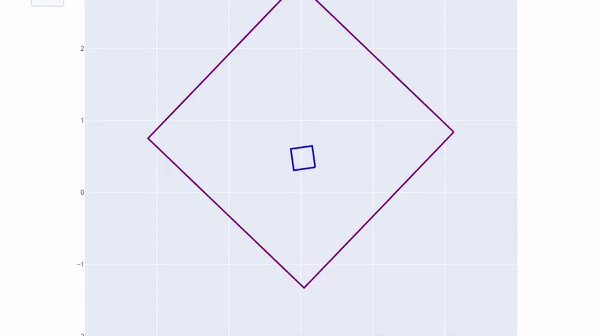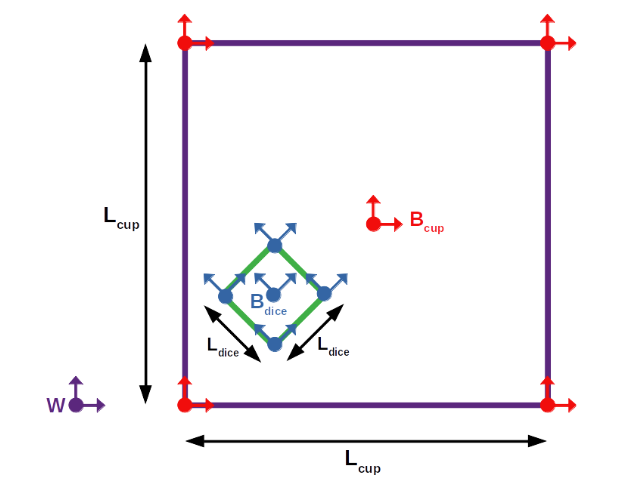# Physics Simulator

Simulated a 2D multi-body system with external forces and impacts using Lagrangian dynamics.## Overview

This project aimed to simulate the motion and impacts associated with a 2D multi-body system of a dice bouncing around inside of a cup. After defining the relevant spatial frames, the Lagrangian equation was calculated using the kinetic and potential energies of the system, taking into account the inertial properties of each body. With the Lagrangian equation, the Euler-Lagrange equations were then calculated. Finally, impact conditions were defined based on the frames of the system and these conditions continuously checked during the simulation to determine the appropriate physical response.A diagram of the system and the various frames and dimensions associated with it.